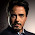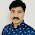For any corporate training requirements Please mail To sudhakar@qtpsudhakar.com
Course Name Date Time Duration Type Location
Selenium Framework Development 22nd July 2019 6.30 AM IST to 8:30 AM IST 15 Days WeekDays Online

A book "Cracking the QTP interview" authored by Sudhakar.Read More. Buy From FlipKart

Thursday, September 23, 2010

Excel Object Model Samples

The below Excel Object Model samples are asked in the interviews of SoftBrand Solutions,Cognizant,IBM,CapGemini,HP and Patni.The Samples are given by Raj Alekapalli. Email: raj4m1983@gmail.com
_______________________________________
Please send your Suggestions and Doubts to my yahoo group http://in.groups.yahoo.com/group/qtpsudhakar

Create excel file and enter some data save it
'###############################################
'Create excel file and enter some data save it
'###############################################

'Create Excel Object
Set excel=createobject("excel.application")

'Make it Visible
excel.Visible=True

'Set the value in First row first column
excel.Cells(1,1).value="testing"

'Save Work Book
workbooks.saveas"D:\excel.xls"

'Close Work Book
workbooks.Close

'Quit from Excel Application
excel.Quit

'Release Variables
Set workbooks=Nothing
Set excel=Nothing
Reading Values from a Specific excel Sheet
'###############################################
' Reading Values from a Specific excel Sheet
'###############################################

'Create Excel Object
Set excel=createobject("excel.application")

'Make it Visible
excel.Visible=True

'Open the Excel File
Set workbook=excel.Workbooks.Open("D:\excel.xls")

'Get the Control on Specific Sheet
Set worksheet1=excel.Worksheets.Item("Sheet1")

' Display the Values
Msgbox  worksheet1.cells(1,1).value

'Close Work Book
workbook.Close

'Quit from Excel Application
excel.Quit

'Release Variables
Set worksheet1=Nothing
Set workbook=Nothing
Set excel=Nothing
Deleting Rows from Excel Sheet
'###############################################
' Deleting Rows from Excel Sheet
'###############################################

'Create Excel Object
Set excel=createobject("excel.application")

'Make it Visible
excel.Visible=True

'Open the Excel File
Set workbook=excel.Workbooks.Open("D:\excel.xls")

'Get the Control on Specific Sheet
Set worksheet1=excel.Worksheets.Item("Sheet1")

'Delete Row1
worksheet1.Rows("1:1").delete

'Save Excel
workbook.SaveAs("D:\excel.xls")

'Close Work Book
workbook.Close

'Quit from Excel Application
excel.Quit

'Release Variables
Set worksheet1=Nothing
Set workbook=Nothing
Set excel=Nothing
Add and Delete ExcelSheet
'###############################################
' Add and Delete ExcelSheet
'###############################################

'Create Excel Object
Set excel=createobject("excel.application")

'Make it Visible
excel.Visible=True

'Open Existing Excel File
Set workbook=excel.Workbooks.Open("D:\excel.xls")

'Assign a Name
newsheet.name="raj"

'Delete Sheet
Set delsheet=workbook.Sheets("raj")
delsheet.delete

'Close Work Book
workbook.Close

'Quit from Excel Application
excel.Quit

'Release Variables
Set newsheet=Nothing
Set delsheet=Nothing
Set workbook=Nothing
Set excel=Nothing
Copy an Excel Sheet of one Excel File to another Excel File
'###############################################
' Copy an Excel Sheet of one Excel File to another Excel File
'###############################################

'Create Excel Object
Set excel=createobject("excel.application")

'Make it Visible
excel.Visible=True

'Open First Excel File
Set workbook1=excel.Workbooks.Open("D:\excel1.xls")

'Open Second Excel File
Set workbook2=excel.Workbooks.Open("D:\excel2.xls")

'Copy data from first excel file sheet
workbook1.Worksheets("raj").usedrange.copy

'Paste Data to Second Excel File Sheet
workbook2.Worksheets("Sheet1").pastespecial

'Save Workbooks
workbook1.Save
workbook2.Save

'Close Workbooks
workbook1.Close
workbook2.Close

'Quit from Excel Application
excel.Quit

'Release Variables
Set workbook1=Nothing
Set workbook2=Nothing
Set excel=Nothing
Comapre Two Excel Sheets Cell By Cell for a specific Range
'###############################################
' Comapre Two Excel Sheets Cell By Cell for a specific Range
'###############################################

'Create Excel Object
Set excel=createobject("excel.application")

'Make it Visible
excel.Visible=True

'Open Excel File
Set workbook=excel.Workbooks.Open("D:\excel.xls")

'Get Control on First Sheet
Set sheet1=excel.Worksheets.Item("Sheet1")

'Get Control on Second Sheet
Set sheet2=excel.Worksheets.Item("Sheet2")

'Give the specific range for Comparision
CompareRangeStartRow=1
NoofRows2Compare=4
CompareRangeStartColumn=1
NoofColumns2Compare=4

'Loop through Rows
For r=CompareRangeStartRow to(CompareRangeStartRow+(NoofRows2Compare-1))

'Loop through columns
For c=CompareRangeStartColumn to(CompareRangeStartColumn+(NoofColumns2Compare-1))

'Get Value from the First Sheet
value1=Trim(sheet1.cells(r,c))
'Get Value from the Second Sheet
value2=Trim(sheet2.cells(r,c))

'Compare Values
If value1<>value2 Then

' If Values are not matched make the text with Red color
sheet2.cells(r,c).font.color=vbred

End If

Next

Next

'Save workbook
workbook.Save

'Close Work Book
workbook.Close

'Quit from Excel Application
excel.Quit

'Release Variables
Set sheet1=Nothing
Set sheet2=Nothing
Set workbook=Nothing
Set excel=Nothing
Reading complete data from excel file
'###############################################
' Reading complete data from excel file
'###############################################

'Create Excel Object
Set excel=createobject("excel.application")

'Make it Visible
excel.Visible=True

'Open Excel File
Set workbook=excel.Workbooks.Open("D:\excel.xls")

'Get Control on Sheet
Set worksheet=excel.Worksheets.Item("raj")

'Get the count of used columns
ColumnCount=worksheet.usedrange.columns.count

'Get the count of used Rows
RowCount=worksheet.usedrange.rows.count

'Get the Starting used Row and column
top=worksheet.usedrange.row
lft=worksheet.usedrange.column

'Get cell object to get the values cell by cell
Set cells=worksheet.cells

'Loop through Rows
For row=top to (RowCount-1)
rdata=""
'Loop through Columns
For col=lft to ColumnCount-1
'Get Cell Value
word=cells(row,col).value

'concatenate all row cell values into one variable
rdata=rdata&vbtab&word
Next

'Print complete Row Cell Values
print rdata
Next

'Close Work Book
workbook.Close

'Quit from Excel Application
excel.Quit

'Release Variables
Set worksheet=Nothing
Set workbook=Nothing
Set excel=Nothing
Read complete data from an Excel Sheet content
'###############################################
' Read complete data from an Excel Sheet content
'###############################################

'Create Excel Object
Set excel=createobject("excel.application")

'Make it Visible
excel.Visible=True

'Open Excel File
Set workbook=excel.Workbooks.open("D:\excel.xlsx")

'Get Control on Sheet
Set worksheet=excel.Worksheets.Item("Sheet1")

'Get Used Row and Column Count
rc=worksheet.usedrange.rows.count
cc=worksheet.usedrange.columns.count

'Loop through Rows
For Row=1 to rc
'Loop through Columns
For Column=1 to cc
'Get Cell Data
RowData=RowData&worksheet.cells(Row,Column)&vbtab
Next
RowData=RowData&vbcrlf
Next

'Display complete Data
msgbox RowData

'Close Work Book
workbook.Close

'Quit from Excel Application
excel.Quit

'Release Variables
Set worksheet=Nothing
Set workbook=Nothing
Set excel=Nothing
Assign Colours to Excel Sheet Cells, Rows
'###############################################
' Assign Colours to Excel Sheet Cells, Rows
'###############################################

'Create Excel Object
Set excel=createobject("excel.application")

'Make it Visible
excel.Visible=True

'Add a New work book

'Get the Excel Sheet
Set worksheet=excel.worksheets(1)

'Coloring Excell Sheet Rows
Set objrange=excel.activecell.entirerow
objrange.cells.interior.colorindex=37

'Coloring Excell Sheet Cell
worksheet.cells(2,1).interior.colorindex=36

'Save Excel
workbook.SaveAs("D:\excel.xls")

'Close Work Book
workbook.Close

'Quit from Excel Application
excel.Quit

'Release Variables
Set objrange=Nothing
Set worksheet=Nothing
Set workbook=Nothing
Set excel=Nothing

1.Heay,

Have you ever tried validation of excel data based on the color of cell ? Suppose when we are comparing two excel sheets cell wise, Once the data is matched in the second sheet, we change the color of it as Green(Which signifies that the data is matched) Second time when you try to compare the another cell content of first sheet with one by one cell content of sheet2, it should skip the already found ( changed into Green color)data and continue to compare with rest of the cells data.
Could you also please include it?

1.its very simple one!
in the loop jsut add a condition that will check cell's interior color
if the color is green(i.e already matched) then skip that pass and continue with next itration of your loop.

thank you

venkatesh
feel free to call me at any time
9158220044

2.Realy great

3.Very very useful. Thanks a lot...

4.5.Very useful. Keep publishing more, plz.

6.7.Very help full...ThanQ..
and I have One Doubt..
How to find the Used range of 2nd column in Excel Sheet?(excel sheet having 2 or more Columns and rows used range also different)

8.How can vbscript get the value of an Excel combo box on a sheet (not on a form)?

9.I am facing error as "Object not found" for Windows("Microsoft Excel").Activate

10.in excel sheet in first column needs to print index, in second column needs to fill with color and in third column need to print color filled if the previous column

11.Hi Could you please let me know different ways to read excel in QTP. I know 1st)Importing Excel to Data table and 2nd Directly reading from excel sheet. Is there any other methods. Qns asked in Iron mountain and HCL technologies

1.There are three ways
1) Datatable Import method
2) Excel Object Model ethod
3) Adodb Method (Read excel as DB method)